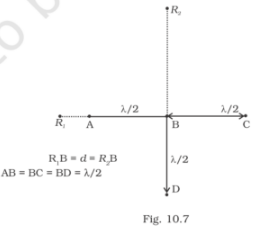# Four identical monochromatic sources A, B, C, D

Question:

Four identical monochromatic sources A, B, C, D as shown in the figure produce waves of the same wavelength λ and are coherent. Two receiver R1 and R2 are at great but equal distances from B.

(i) Which of the two receivers picks up the larger signal?

(ii) Which of the two receivers picks up the larger signal when B is turned off?

(iii) Which of the two receivers picks up the larger signal when D is turned off?

(iv) Which of the two receivers can distinguish which of the sources B or D has been turned off?Solution:

(i) R2 is the larger signal.

(ii) When B is switched off, R1 is y = a cos ꞷt

IR1 = a2/2

R2 is y = a cos ꞷt

IR2 = a2/2

So, it can be said that R1 and R2 are the same.

(iii) When D is switched off, R1 is y = a cos ꞷt

IR1 = 1/2 a2

R2 is y = 3a cos ꞷt

IR2 = 9a2/2

(iv) Therefore, R1 indicates B when B is switched off and R2 indicates D is switched off.